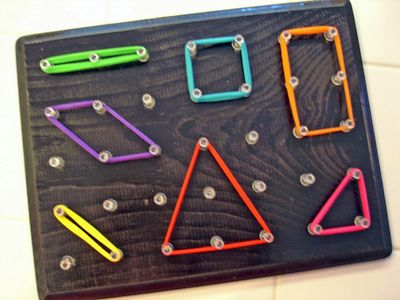# Grade 2: Math: Resources and Refreshers for Parents & Families

A collection of activities and resources, chosen to help support the Ontario, Grade 2, math curriculum. Includes resources for teacher and parents!

## Ontario Math Curriculum## More Fun and Games!## ♥ ♥ ♥ Math!

Grade 2 is a fun year for Math! Here’s what they will be learning over the course of the year:

- Number Sense and Numeration

A student will learn numbers to 100, money amounts to 100¢, decomposing and composing two-digit numbers, and investigating fractions of a whole. They will learn to count by 1’s, 2’s, 5’s, 10’s, and 25’s, adding and subtracting two-digit numbers in a variety of ways, begin multiplying and dividing.

- Measurement

A student will begin measuring length using centimetres and metres, and telling time to the nearest quarter-hour. Learn how to measuring perimeter, area, mass, and capacity using non-standard units and describing and establishing temperature change. They will find personal referents for the centimetre and the metre, compare mass and capacity using non-standard units, and begin relating days to weeks and months to years.

- Geometry and Spatial Sense

A student will start distinguishing between attributes that are geometric properties and attributes that are not geometric properties, learn classifying two-dimensional shapes by geometric properties (number of sides and vertices) and classifying three-dimensional figures by geometric properties (number and shape of faces). The will learn locating a line of symmetry, composing and decomposing shapes, and be able to describe relative locations and paths of motion.

- Patterning and Algebra

Students will be able to identify and describe repeating patterns and growing and shrinking patterns. They will develop the concept of equality using the addition and subtraction of numbers to 18 and the equal sign, and learn the property of zero in addition to facilitate computation

- Data Management and Probability

A student will learn how to organize objects into categories using two attributes. They will be able to collect and organize data and learn reading and displaying data using line plots and simple bar graphs. The will learn to describing probability, in simple games and experiments, as the likelihood that an event will occur.

## Help! I can't remember how to do that!

Refreshers for parents helping with with homework. For lots of how-to videos,